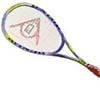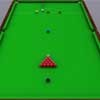#### You may also like### Rain or Shine

Predict future weather using the probability that tomorrow is wet given today is wet and the probability that tomorrow is wet given that today is dry.### Squash

If the score is 8-8 do I have more chance of winning if the winner is the first to reach 9 points or the first to reach 10 points?### Snooker

A player has probability 0.4 of winning a single game. What is his probability of winning a 'best of 15 games' tournament?

# Dicey Dice

##### Age 16 to 18Challenge Level

Steve writes this about his problem:

We will need to use a mixture of common sense and conditional probability to solve this problem, as there is no obvious systematic point to get started with this anaysis. The key equation we need is:
$$P(A> B) = P(A> B|A=1)P(A=1)+\dots +P(A> B|A=6)P(A=6)$$
An obvious starting point would be to consider the case where dice B has only one number on it, 3, say.

For A to have a greater than 50% chance to beat B, A must have 4 higher numbers, Say 4444xx.

For B to have greater than 50% chance to beat C, C must have 4 lower numbers, say xx2222. Can C beat A? If C = 662222 and A = 444411 then P(C beats A) = 1/3 x 1 + 2/3 x 1/3 = 5/9

To find dice with equal probabilities after a bit of fiddling around we settle on the answer 5/9. The trick is to adjust down any dice which are too strong and up any dice which are too weak. There is some flexibility to adjust numbers without altering the probabilities for any other wins/losses.

$$\begin{eqnarray} P(A> B) &=& P(A> B|A=5)\times \frac{1}{3}+P(A> B|A=4)\times \frac{1}{3}+P(A> B|A=1)\times \frac{1}{3}\\ &=&1\times \frac{1}{3}+ \frac{2}{3}\times \frac{1}{3}+0\times\frac{1}{3}\\ &=& \frac{4}{9} \end{eqnarray}$$